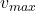A toy is undergoing SHM on the end of a horizontal spring with force constant 310 N/m . When the toy is 0.120 m from its equilibrium positio

Question

A toy is undergoing SHM on the end of a horizontal spring with force constant 310 N/m . When the toy is 0.120 m from its equilibrium position, it is observed to have a speed of 3 m/s and a total energy of 3.6 J .
1.) Find the mass of the toy.
2.) Find the amplitude of the motion.
3.) Find the maximum speed obtained by the object during its motion.

in progress 0
6 months 2021-08-04T07:19:45+00:00 1 Answers 21 views 0

1) the mass of the toy is 0.304 kg

2) the amplitude of the motion is 0.1524 m

3) the maximum speed obtained by the object during its motion is 4.87 m/s

Explanation:

Given the data in the question;

force constant of spring k = 310 N/m

position of toy x = 0.120 m

speed v = 3 m/s

Total energy E = 3.6 J

let m represent mass of toy.

1.) Find the mass of the toy.

we know that; The total energy of the system equals the sum of kinetic energy of the toy and the potential energy of the spring;

E =kx² +mv²

we substitute

3.6 = (× 310 × (0.120)² ) + (× m × (3)² )

3.6 = 2.232 + 4.5m

3.6 – 2.232 = 4.5m

1.368 = 4.5m

m = 1.368 / 4.5

m = 0.304 kg

Therefore, the mass of the toy is 0.304 kg

2) Find the amplitude of the motion.

we know that;

E =kA²

where A is the amplitude of the motion,

we substitute

3.6 =× 310 × A²

3.6 = 155 × A²

A² = 3.6 / 155

A² = 0.0232258

A = √0.0232258

A = 0.1524 m

Therefore, the amplitude of the motion is 0.1524 m

3) Find the maximum speed obtained by the object during its motion;

we know that;

E =m²

whereis the maximum speed

so we substitute

3.6 =× 0.304 ×²

3.6 = 0.152 ×²² = 3.6 / 0.152² = 23.6842² = √23.6842² = 4.8664 ≈ 4.87 m/s

Therefore, the maximum speed obtained by the object during its motion is 4.87 m/s